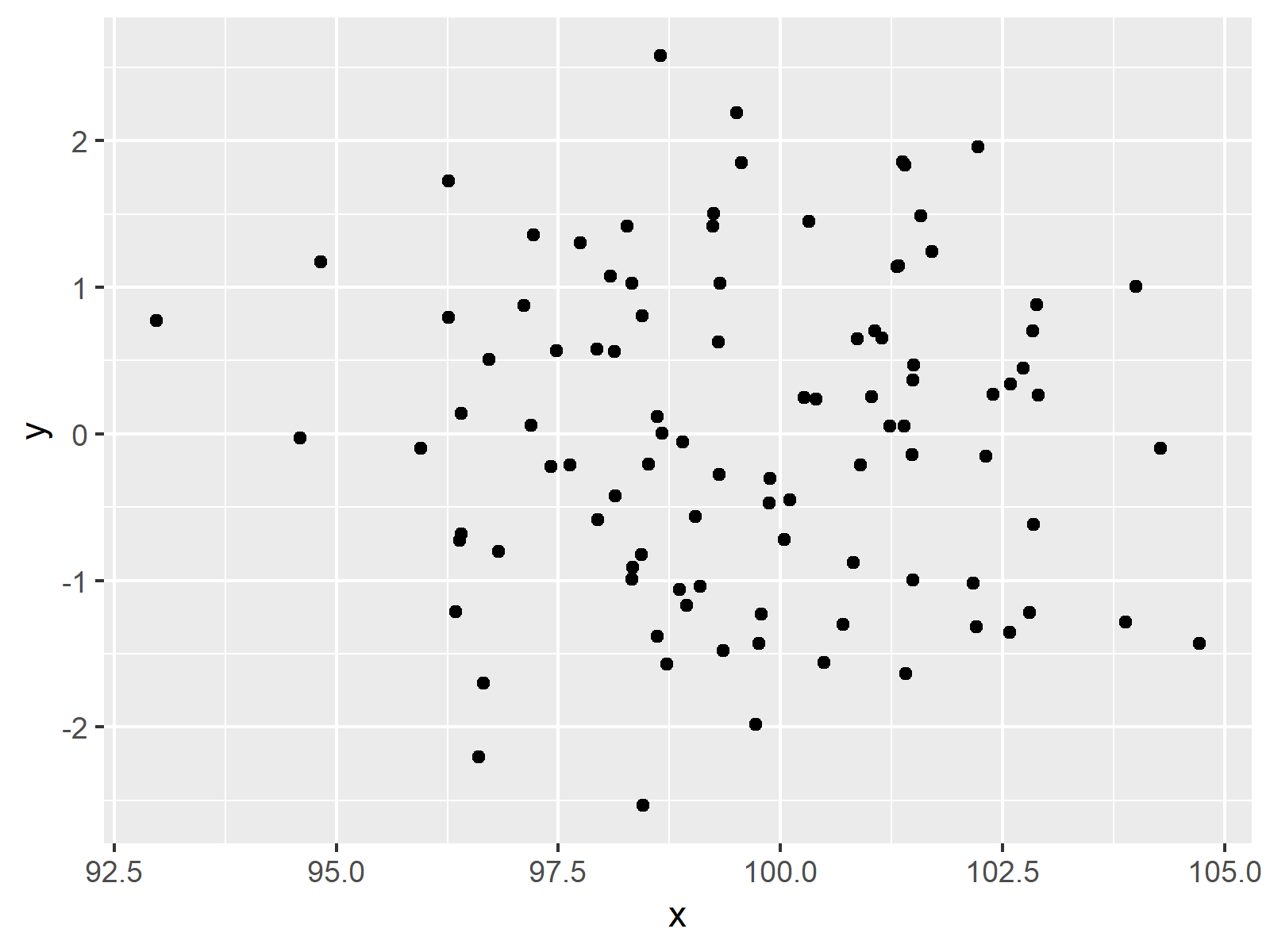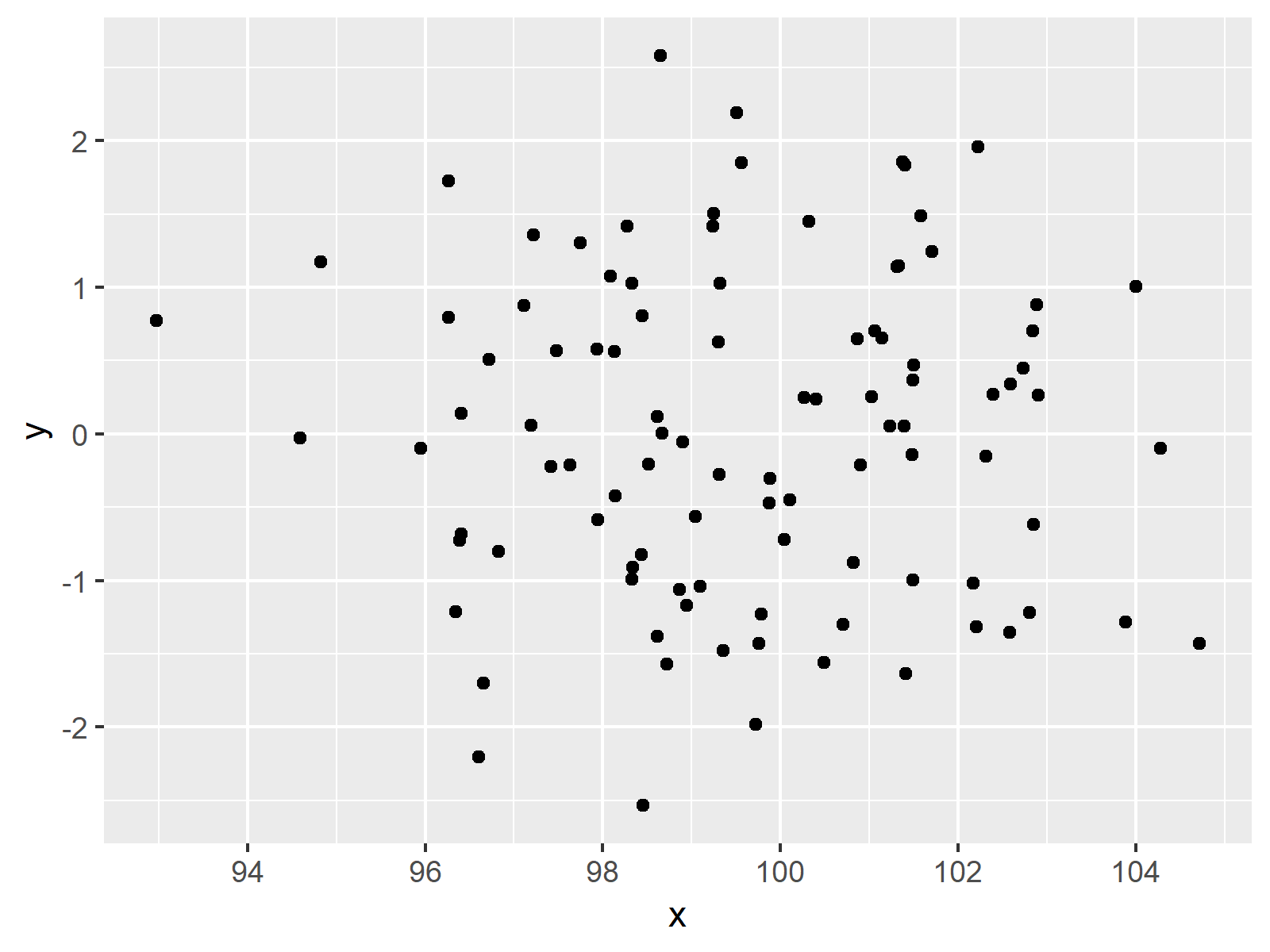# Display Only Integer Values on ggplot2 Axis in R (Example)

In this tutorial you’ll learn how to draw a ggplot2 axis containing only integers in R.

The content of the page is structured as follows:

Let’s dive right in.

## Example Data, Packages & Default Plot

First, we’ll have to create some data that we can use in the examples later on:

```set.seed(10821)                     # Create data
data <- data.frame(x = rnorm(100, 100, 2),
y = rnorm(100))
#           x           y
# 1 100.31996  1.45011641
# 2 101.13881  0.65336914
# 3  96.82407 -0.80131958
# 4 100.10791 -0.44847950
# 5  94.59277 -0.02716243
# 6 101.58426  1.48654344```

As you can see based on the previous output of the RStudio console, our example data consists of two columns both containing random numeric values.

If we want to create a plot of our data with the ggplot2 add-on package, we also have to install and load ggplot2:

```install.packages("ggplot2")         # Install & load ggplot2
library("ggplot2")```

Next, we can plot our data:

```ggp <- ggplot(data, aes(x, y)) +    # Create default ggplot2 graph
geom_point()
ggp                                 # Print plot```The output of the previous code is shown in Figure 1 – A default ggplot2 plot with non-integer values on the x-axis.

## Example: Display Prettier Axis Values Using scales Package

This Example explains how to remove non-integer numbers from ggplot2 axes in R. First, we have to install and load the scales package:

```install.packages("scales")         # Install & load scales
library("scales")```

Now, we can use the pretty_breaks function of the scales package to modify our axis values.

```ggp +                               # Improve scales
scale_x_continuous(breaks = pretty_breaks())```As shown in Figure 2, the previous syntax created a ggplot2 scatterplot showing only integer numbers as axis breaks.

## Video & Further Resources

Would you like to know more about the ggplot2 package? Then you may have a look at the following video of my YouTube channel. I explain how to use the ggplot2 package in much more detail:

Please accept YouTube cookies to play this video. By accepting you will be accessing content from YouTube, a service provided by an external third party.If you accept this notice, your choice will be saved and the page will refresh.

Besides that, you may read the other tutorials of my website.

At this point you should know how to create a ggplot2 axis with only integer values in the R programming language. Let me know in the comments, if you have further questions.

Subscribe to the Statistics Globe Newsletter